# Volume of Revolution Discussion i

The formula for finding a volume of a solid formed by revolving a smooth and continuous functionwhere, 360° around theaxis isLet’s find out why. Here’s the outline of the discussion:

1. Examine the simple case,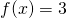.
2. Agree that the functionexists.
3. Agree that ifis smooth and continuous, thenis smooth and continuous.
4. Conclude thatwhich leads to# Examine a simple case

Let’s take, fromto.

By rotating the straight, horizontal linearound theaxis, we generate a cylinder. We already know that the volume of a cylinder can be found using the formula:Whereis the radius of the cross sectional circle, andis the length or height of the cylinder.

We also remember that a function maps a value from one set (domain) to another set (range). Let’s create a functionthat maps the length of the cylinder to the volume of the cylinder. Our domain is the length of the cylinder, which in our case is the measurement on theaxis. If we keep one end of the cylinder parked at, then the length of the cylinder is just.

The cylinder length. The cylinder radius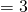. Using the volume of cylinder formula given above, our volume function is:We see that this agrees withwhenas follows:Let’s check out the volume of the cylinder whenand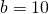, using our function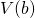:Now let’s check out the volume of the cylinder whenand.Lastly, let’s check out the volume of the cylinder whenand. We can calculate this by taking the longer cylinder (from zero to ten) and subtracting the shorter cylinder (from zero to 4).The calculation above is exactly the calculation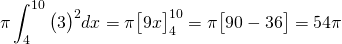Let’s move on to understand why this calculation works for any smooth, continuous function, not just the simple straight line that generates the familiar cylinder.

Next step: Agree that the functionexists for other smooth continuous functions. (under construction)